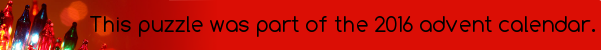mscroggs.co.ukmscroggs.co.uksubscribe

# Puzzles

## 23 DecemberToday's number is the area of the largest area rectangle with perimeter 46 and whose sides are all integer length.

## 12 DecemberThere are 2600 different ways to pick three vertices of a regular 26-sided shape. Sometime the three vertices you pick form a right angled triangle.These three vertices form a right angled triangle.
Today's number is the number of different ways to pick three vertices of a regular 26-sided shape so that the three vertices make a right angled triangle.

## Equal lengths

The picture below shows two copies of the same rectangle with red and blue lines. The blue line visits the midpoint of the opposite side. The lengths shown in red and blue are of equal length.
What is the ratio of the sides of the rectangle?

## Is it equilateral?

In the diagram below, $$ABDC$$ is a square. Angles $$ACE$$ and $$BDE$$ are both 75°.
Is triangle $$ABE$$ equilateral? Why/why not?

## Bending a straw

Two points along a drinking straw are picked at random. The straw is then bent at these points. What is the probability that the two ends meet up to make a triangle?

## Placing plates

Two players take turns placing identical plates on a square table. The player who is first to be unable to place a plate loses. Which player wins?

## 20 DecemberEarlier this year, I wrote a blog post about different ways to prove Pythagoras' theorem. Today's puzzle uses Pythagoras' theorem.
Start with a line of length 2. Draw a line of length 17 perpendicular to it. Connect the ends to make a right-angled triangle. The length of the hypotenuse of this triangle will be a non-integer.
Draw a line of length 17 perpendicular to the hypotenuse and make another right-angled triangle. Again the new hypotenuse will have a non-integer length. Repeat this until you get a hypotenuse of integer length. What is the length of this hypotenuse?

## 17 DecemberThe number of degrees in one internal angle of a regular polygon with 360 sides.

## Archive

Show me a random puzzle
▼ show ▼